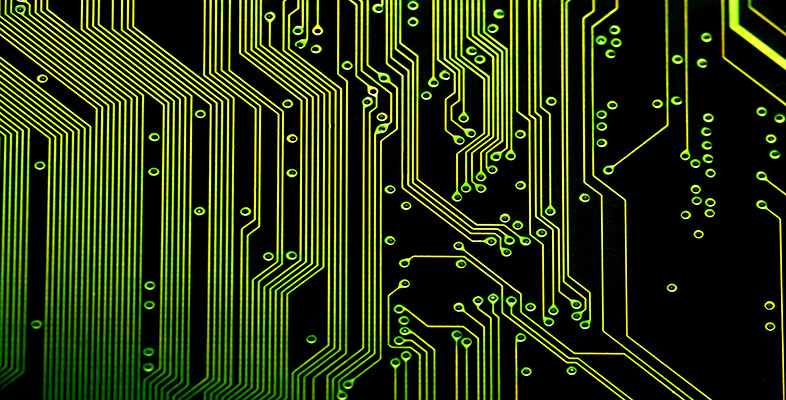Science, Maths & Technology

### Become an OU studentIntroduction to computational thinking

Start this free course now. Just create an account and sign in. Enrol and complete the course for a free statement of participation or digital badge if available.

# 2.6 Varieties of abstraction

In the next video clip, Wing compares and contrasts abstraction in computational thinking with abstraction in mathematics and engineering. In the following activity you will make these comparisons explicit.

## Activity 6 Computational, mathematical and engineering thinking

Timing: 20 minutes

### Parts 1-8

Watch the video and complete the activity by ticking the appropriate boxes.

Download this video clip.Video player: Computational, mathematical and engineering thinking
Computational, mathematical and engineering thinking
Interactive feature not available in single page view (see it in standard view).

### Part 1

1. Which of the following use formal notation?

a.

Computational thinking

b.

Mathematical thinking

c.

Engineering thinking

The correct answers are a, b and c.

#### Discussion

In all three disciplines, the use of formal/mathematical notation is common:

### Part 2

2.Which of the following involve at least two layers?

a.

Computational thinking

b.

Mathematical thinking

c.

Engineering thinking

The correct answers are a and c.

#### Discussion

Mathematics typically works only with a single layer: there is no distinction between the ‘properties of interest’ and the ‘ignored detail’. The abstraction is studied in its own right, without reference to some other layer which it represents. Of course, this is an idealisation. Many areas of mathematics did start as a representation of some aspect of the real world. For example, geometry began with Euclidean geometry, which was intended as a model of physical space.

Both engineering and computing rely on at least two layers. At the basis, there is a layer grounded in the real world. This is the layer in which the problem of interest lies (for example a bridge or car in engineering). In addition to this layer, there is at least one further layer which ignores certain of its details. For instance, an engineer may use mechanics to calculate the forces operating on a bridge when a car crosses it. In doing so, the engineer will abstract away (or ignore), the colour in which the bridge is painted, the brand of the car, and many other things that are not relevant for calculating the forces in question. We’ve seen that a computer scientist who simulates a dictionary may, similarly, ignore certain properties of a physical dictionary.

### Part 3

3. For which of the following are beauty and elegance measures of goodness?

a.

Computational thinking

b.

Mathematical thinking

c.

Engineering thinking

The correct answers are a, b and c.

#### Discussion

In all three disciplines, beauty and elegance are criteria that are used to compare competing abstractions. These are, however, more central in mathematics, where the abstraction is often judged in its own right, without reference to an underlying reality that it represents.

### Part 4

4. For which of the following is correctness a measure of goodness?

a.

Computational thinking

b.

Mathematical thinking

c.

Engineering thinking

The correct answers are a, b and c.

#### Discussion

In connection with this criterion, Wing asks in particular: ‘Does it do the right thing?’ and ‘Does it do anything?’ These questions are clearly relevant for both computational and engineering abstractions. It is perhaps less clear what they mean in the context of mathematics. Arguably, since mathematics is about the abstraction itself, one can’t really distinguish correct from incorrect abstractions because there is nothing to compare them with. However, even when dealing with pure abstractions, we may want to make sure that they are consistent. A mathematical theory that states that a mathematical object both does and does not have some property would be in violation of this criterion (e.g. a theory which says that two lines cross and do not cross each other).

### Part 5

5. For which of the following is efficiency a measure of goodness?

a.

Computational thinking

b.

Mathematical thinking

c.

Engineering thinking

The correct answers are a and c.

#### Discussion

In connection with computing, Wing mentions three dimensions of efficiency: time (how fast?), space (how much space?) and energy (how much power?). Each of the three efficiency criteria can also be applied in engineering contexts (e.g. how fast can the engine run, how much space does it occupy, and how much energy does it consume?). None of these considerations has a clear equivalent in mathematics.

### Part 6

6. For which of the following are usability, modifiability, maintainability and cost measures of goodness?

a.

Computational thinking

b.

Mathematical thinking

c.

Engineering thinking

The correct answers are a and c.

#### Discussion

All of these practical considerations are important in both engineering and computing contexts. They are not directly applicable to mathematics.

### Part 7

7. Which of the following are constrained by physics?

a.

Computational thinking

b.

Mathematical thinking

c.

Engineering thinking

The correct answers are a and c.

#### Discussion

Both engineering and computing are constrained by physical reality. For example, the classical model of computing uses the bit (0 or 1) as the basic unit of information. It is constrained by whichever physical means is used to represent a bit (whether it be an electrical current or, for instance, the position of a mechanical lever). The physical hardware constrains, among other things, how quickly the state of a bit can be changed (from 0 to 1 or vice versa).

### Part 8

8. Which of the following can go beyond physics?

a.

Computational thinking

b.

Mathematical thinking

c.

Engineering thinking

The correct answers are a and b.

#### Discussion

Mathematics, as the study of pure abstractions, is independent of physical reality. Computing, though always constrained by the physical layer at which the hardware operates, allows us to build on top of the hardware layer software layers that model non-existent realities. By automating these virtual realities, it allows us to see and even experience realities that go beyond what is physically possible.